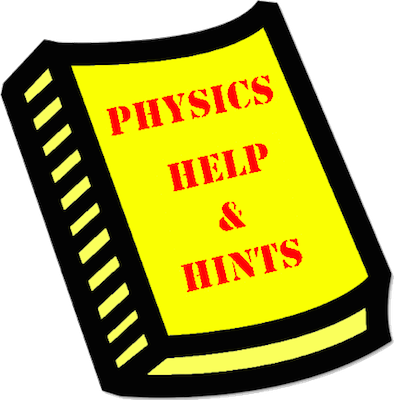# Mission EC11: Combination Circuits Calculations

Mission EC11 pertains to the mathematical analysis of combination circuits. The mission consists of 20 questions organized into 5 Question Groups. You must answer one question from each Question Group to complete the mission. The learning outcomes for this mission are ...

#### Learning Outcomes

• The student should be able to mathematically analyze a combination circuit to determine the equivalent resistance, the total circuit current and the current through each resistor (if given the battery voltage and the resistance of each resistor).

### Launch Mission EC11

#### Getting Help

If you are not familiar with this topic, then you should first learn about the topic using our written Tutorial or our Video Tutorial:

The Physics Classroom, Circuits Unit, Lesson 4, Part e

#### Video Tutorials:

Combination Circuits

####Question-Specific Help

Each Question Group has its own Help page with information specific to the question. You can access the Help page from within the mission by tapping on the Help Me! icon (textbook). For your convenience, links to those pages are provided below: